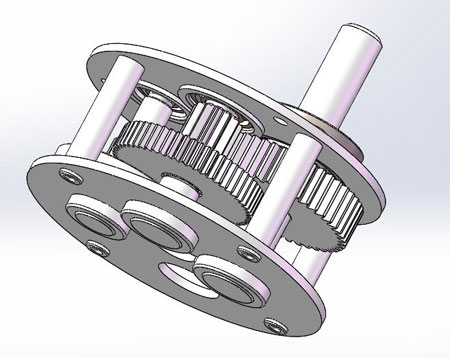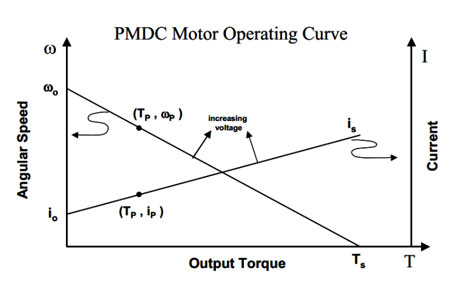Welcome to I.CH Motion Co., Ltd
Email：sales@ichmo.com      Tel：+86-25-51180871

## NEWS CENTRE

You are here: » » How to Select Robot Motor?

# How to Select Robot Motor?

Views:42     Author:xia     Publish Time: 2020-04-03      Origin:SiteIn robots, there are two drive types for actuators. While the motor drive is the most common.

The motors that are used in the robot are brushed DC motor, brushless DC motor, permanent magnet synchronous motor, stepper motor. In small robots, for their low price, high precision, DC motor, and brushless DC motor are widely used.

## Part one: Motor Requirements

The introduction of DC Motor

The choice of motor is often a compromise between our requirements for motor performance and price. So, in the selection process of the motor, we should consider the various parameter of the motor. According to the important parameters that are very important to us, to select motors.

From a motor, it has multiple performance curves. Here, we will list the most important characteristic. From the perspective of the robot drive, our main concerns are:

1, Working voltage:

For a motor, there may be multiple voltage parameters; the most commonly used is the rated voltage under continuous operation conditions. Some motor can operate beyond rated voltage and speed, but after a period of operation, the local overheating problem will occur. That is to say, over-voltage can be operated for a short time, not for a long time.

2, Rotational speed:

The speed of motor rotation, expressed as in revolution per minute, sometimes expressed in radians per second or angles per second;

3, Torque:

The ability to change the rotation speed; for example, when using a wrench to turn the screw, the torque of wrench makes the screw rotate. In the field of robots, torque is used to make the robot move or make the arm of the robot execute different actions; Torque is equal to force multiplied by force arm, whose the unit is Nm.

4, Current:

For a motor, there probably exist multiple current parameters, such as no-load current, rated current, stalled current.

5, Physical parameter:

Such as the size of the motor, the size of the shaft, the size of the section and the location of the fixing hole.

DC Gear Motor:

The current of DC Motor is DC power, So, it can be powered by batteries, which is a reason why DC motors are widely used in robots; there may be the difference in size, but the parameter is generally the same; The direction of DC Motor can be changed by the sign of supply voltage;

Small DC motors usually run in high speed and low torque, which is contrary to the motor drive requirements in robots which require low rpm and high torque. So, to reduce the speed and increase torque, a shaft is added between the motor and output shaft; by the combination of different gearboxes, the motor can obtain different rated speeds and torques. At present, many motors have a gearbox when they are manufactured, it is called a DC gear motor.

The advantages of the gear motor are: easy to use, high output torque, low speed, and a wide range of options. The main disadvantage is the low accuracy. Even the same batch of gear motor, using the same current or voltage, the output of gear motor may be different; therefore, in the application of robot, the control of gear motor should introduce closed-loop control instead of open-loop control.

Here are some common factors to consider when selecting a gear motor:

(1) Versatility:

Generally speaking, the more versatile the motor is, the cheaper it will be.

(2) Working voltage:

Commonly, the working voltage range of small gear motor is 6-24V.

(3) Output torque:

The output torque of the miniature gear motor is between 0.1-0.5Nm; it can be used to drive a robot between 20-30kg.

(4) Rotational speed:

The speed of the motor and the size of the tire will determine the maximum operating speed of the robot; the size of the tire is between 5-20cm.

Although most gear motors can be reversed, some motors can only rotate in one direction. Besides, gear motors have many parameters, but from the perspective of robots, many parameters such as inertia are not concerned. The most important parameters for gear motor in robots are motor speed, torque, and rated voltage.

Example:

Brand: I.CH Installation: horizontal type

Stage: Level 3 Tooth surface hardness: Hard tooth surface

Layout: coaxial

Gear ratio: 103 Rated voltage: 24V

Rated speed: 131Rpm No-load speed: 155Rpm

Rated Torque: 0.014Nm Peak torque: 0.075Nm

Motor size:

Motor diameter:20mm Motor length: 45.5mm

Output shaft diameter: 4mm

Output shaft step height: 0.5mm

Output shaft length: 11mm

Motor parameter requirements:

First of all, we need to determine the working environment such as whether robots run outdoors or indoors, whether the surface of the land is smooth or rough, whether climbing or not. Secondly, we need to determine the specific parameters of robots, such as size, weight, etc. Then we need to running parameters, such as speed and torque.

The motors drive the robot tires to make the robot move. The speed of the robot mainly depends on motor speed and the diameter of the tire. The rotation speed of the motor is based on the voltage and its load torque. At the condition of the same supply voltage, the rotation of speed under no load is higher than the rotation speed under load. At the same supply voltage, the greater the output power of the motor, the lower the motor speed, the greater the motor current. When the output power reaches up to a certain level, the motor will stop rotating, this is stall. At this time, the motor current is the largest.

The relationship between robot tire speed and motor rotation speed:

V=0.02*pi*w*R/60;

V is the running speed of the robot(m/s).

W is the motor rotation speed(rpm); r is the motor radius(cm)

Suppose we require the average speed of the robot is Vaag, the speed of the gear motor is:

W=60*Vaag/(0.02*pi*R) (1)

Motor torque requirements:

The motor torque requirements are related to ground friction and slope.

Assume that the friction coefficient is C, the weight is m(kg), the torque which is required for the robot to run at a uniform speed on the ground is:

T=0.01Ff*R=0.01C*mg*R;

F is the friction (N)

In addition to uniform motion, we may also specify the maximum climbing angle theta and maximum acceleration a. At this time, the two can be equivalent to friction:

C=C0+sin(theta)+a/g;

C0 is the inherent ground friction coefficient

Theta is the maximum climbing

A is the maximum acceleration

G is the acceleration of gravity.

T=0.01CmgR (2)

The above formulas 1 and 2 are the method of selecting the speed and torque of the robot motor.

## Part two: Motor operating conditions

When DC motor starts to supply power, the motor shaft has not yet to rotate. At this time, the motor is in a stalled status. The current is iS, and the torque generated by the motor is the largest; So the motor starts to rotate. At this time, with the speed increases, the current and torque decrease. When the motor torque is equal to load torque, the motor reaches a balanced status.In the field of robot applications, we are often concerned about the maximum speed that the motor can achieve under different load conditions.

For example, under the no-load conditions, the no-load speed is w0; under load conditions, stall torque is Ts;

If the load torque is T, then, the maximum speed of the motor is:

W=w0*(1-T/Ts) ;

If the maximum speed is w, then the maximum torque is:

T=Ts*(1=w/w0);

When the motor toque is T, the current is:

i=i0+(is-i0)*T/Ts;

iS: stall current

Ts: stall torque

After the above discussion, we can adopt such a method. In the process of selecting the gear motor, we should consider the following parameters:

1, Motor operating voltage:

When selecting the motor operating voltage, we should be based on the voltage of the robot battery. Most motors operate at 6V, 12V, 24V. for robots with a general size of 15-30cm, nickel-hydrogen batteries are usually used. For large robots, lead-acid is usually used; the standard size of nickel-hydrogen batteries are AA, C, D, and the voltage is 3.6V-48V. For 12V motors, we use 7.2V or 9.6V supply power. For 24V motors, we generally use 3*9.6V supply power.

2, Motor speed:

For the estimation of motor speed, we choose motors based on the rated voltage and rated torque. For robots with general tire sizes between 5-20cm, the speed is 40-300rpm.

3, Motor torque:

In the process of selecting motor torque, we leave a 50% margin based on the calculation in the previous section; besides, if the number of motors is more than one, the motor torque requirements can be divided by the corresponding amount.

4, Motor current:

The current will determine the running time of the robot. Based on the current data, we can roughly evaluate its application range. If a motor current is 0.01A, for most robot applications, the motor will be too small. If the rated current of the motor is 1A, then the motor will be large.

Also, you should pay attention to the size of the robot, to ensure that the motor can be installed. Also, according to requirements of control accuracy, to choose a suitable position sensor or rotary transformer.

## Part three: Examples of Robot motor selection

The robot weighs 4kg and requires a maximum running speed of 1m/s. two motors drive it to walk. The maximum climbing angle is 5 degrees, the voltage of the motor is 24V and the tire diameter is 65mm.

1, speed requirement:

Speed=60*Velocity/(PI*d)=290rpm;

2, torque requirement:

Assuming a friction coefficient of 0.03, the torque is:

T=Ff*R=(0.03+sin5)*4kg*9.8kgm/s^2*32.5*10^-3m=100mNm;

Share it to two motors, each motor torque is 50mNm.

Gear motor parameters are:

Torque: 50mNm

Speed: 290rpm

## SUBSCRIBE NOW

We have an independent product design and R&D team, service team and professional quality control team. Welcome to subscribe.
We specialized in researching, developing and servicing electric motors, gearbox and high precision gears with small module. Read more >>